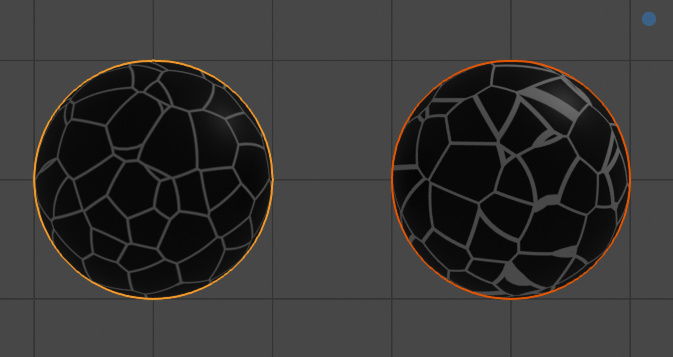# Voronoi distance to edge along surface normal

I’m not sure how useful this is in general but I’ve found myself wanting the Voronoi Texture to give the distance to the closest edge along the surface normal. This is quite a bit different from what the Voronoi Crackle gives out (which incidentally didn’t exist in Cycles when I started looking into this problem.)

I made an OSL script that shows how this edge distance can be calculated, but the gist is shown with this image:The the left is my OSL script taking the distance along the surface normal and the right is Voronoi Crackle. The crackle gives widely varying rate of change on the edges because the Voronoi texture is a volume and some of the faces dividing the cells align with the surface the texture is applied to.

Is this something that would be a good idea to implement in Cycles?

OSL Vornoi edge distance script
``````// Bob Jenkins' 4-byte integer hash, full avalance
// http://burtleburtle.net/bob/hash/integer.html
int hash(int a)
{
// OSL doesn't have hexadicimal, rip sanity
a = (a+ 2127912214) + (a<<12);
a = (a^ -949894596) ^ (a>>19);
a = (a+  374761393) + (a<<5);
a = (a+ -744332180) ^ (a<<9);
a = (a+  -42973499) + (a<<3);
a = (a^-1252372727) ^ (a>>16);
return a;
}

vector cell_position(int x, int y, int z)
{
int h = hash(x&1023 | (y&1023) << 10 | (z&1023) << 20);
return vector (h & 1023, (h>>10) & 1023, (h>>20) & 1023) / 1024;
}

int conv(float v)
{
if (v > 0) {
return int(v);
} else {
return (int(v)-1);
}
}

vector my_faceforward(vector n, vector i, vector nref)
{
return dot(nref, i) < 0 ? n : -n;
}

/*
* Given a vector v and a direction d, calculates the distance needed to travel
* before hitting plane p oriented along normal n
*/
float plane_distance(vector v, normal d, vector p, normal n)
{
return -dot(v-p, n) / dot(d, n);
}

float edge_distance(vector center, vector edge, vector p)
{
vector midpoint = (center+edge) / 2.0;
normal plane = calculatenormal(p);
normal dir = normalize(cross(plane, cross(plane, center-edge)));
return plane_distance(p, dir, midpoint, center-edge);
}

point Vector = P,
float scale = 5.0,
output color Color = color(0,0,0)
) {
vector p = Vector * scale;
int x = conv(p);
int y = conv(p);
int z = conv(p);

vector f1;
float f1d = 10000000000.0;
vector f2;
float f2d = 10000000000.0;
vector f3;
float f3d = 10000000000.0;
vector f4;
float f4d = 10000000000.0;

for (int i = x-2; i <= x+2; ++i) {
for (int j = y-2; j <= y+2; ++j) {
for (int k = z-2; k <= z+2; ++k) {
vector pos = cell_position(i, j, k) + vector (i, j, k);
float d = distance(p, pos);

if (d < f1d) {
f4 = f3;
f4d = f3d;
f3 = f2;
f3d = f2d;
f2 = f1;
f2d = f1d;
f1 = pos;
f1d = d;
} else if (d < f2d) {
f4 = f3;
f4d = f3d;
f3 = f2;
f3d = f2d;
f2 = pos;
f2d = d;
} else if (d < f3d) {
f4 = f3;
f4d = f3d;
f3 = pos;
f3d = d;
} else if (d < f4d) {
f4 = pos;
f4d = d;
}
}
}
}

float f2ed = edge_distance(f1, f2, p);
float f3ed = edge_distance(f1, f3, p);
float f4ed = edge_distance(f1, f4, p);
Color = min(min(f2ed, f3ed), f4ed);
}
``````
6 Likes

Looks like a very useful feature, I would accept a patch adding this to Cycles.

2 Likes

Patch implementing this idea here: https://developer.blender.org/D3673

3 Likes